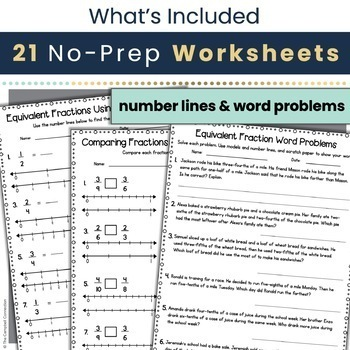# Equivalent Fractions Worksheet on a Number Line | 3rd Grade3rd - 4th, Homeschool
Subjects
Standards
Resource Type
Formats Included
• Zip
Pages
21 pages

#### Also included in

1. This bundle includes all of our third grade Common Core equivalent fractions on a number line activities. It includes task cards, practice worksheet bundle, daily math, Google Classroom™ activities, I Have… Who Has game, and a QR Code scavenger hunt. All of these activities are ready to print and wi
\$42.28
\$52.85
Save \$10.57
2. Teaching 3rd grade math review can be challenging, but it doesn't have to be when you have daily 3rd grade math worksheet activities to support your daily instruction in your third-grade math class. To successfully help your students build a solid foundation, you need engaging and interactive materi
\$37.64
\$47.05
Save \$9.41

### Description

This equivalent fractions activity is an in-depth way to learn how to find equivalent fractions using models, number lines, and word problems. Along with comparing fractions using greater, less than, and equal to. Use this with any 3rd-grade classroom or as a review of examples for equivalent fractions for 4th grade.

The Equivalent fractions worksheets take a scaffolded approach and simplify teaching this difficult standard. Students will go from using models that are already filled in, filling in their own fraction models, to creating their own. The same is done with number lines. The worksheets start with labeled number lines and slowly take components away to support the students in creating their own.

This easy-to-use, best-selling activity includes:

• 3 worksheets using fraction models to show equivalence

• 4 worksheets using fraction number lines to show equivalence

• 2 worksheets using a mixture of fraction models & number lines to show equivalence

• 3 worksheets using fraction models to compare

• 3 worksheets using fraction number lines to compare

• 2 worksheets using a mixture of fraction models & number lines to compare

• 2 worksheets using whole numbers

• 2 worksheets using word problems

Want a FREE sample of this activity? Get it here:

Equivalent Fractions Worksheet 1 Page Sample

Looking for even more? Here are money-saving bundles: Save 20%!

Equivalent Fractions Activities Bundle

There are several ways you can use these equivalent fractions activities:

• Independent practice in class

• Pair and Share activity

• Small group work

• Extra remediation

• Homework

• Test review and practice

• Quiz

• Informal assessment

• Guided math work

• Early finisher work/bonus problems

• Morning work

▪▪▪▪▪▪▪▪▪▪▪▪▪▪▪▪▪▪▪▪▪▪▪▪▪▪▪▪▪▪▪▪▪▪▪▪▪▪▪▪▪▪▪▪▪▪▪▪▪▪▪▪▪▪▪▪▪▪▪▪▪▪▪▪▪▪▪▪▪▪▪▪▪▪▪▪▪▪▪▪▪▪▪▪▪▪▪▪▪▪▪▪▪▪▪▪▪▪▪▪▪▪▪▪▪

Looking for more Equivalent Fraction practice or even digital activities? Check out these activities -

Equivalent Fractions with Models in Google Slides

Equivalent Fractions with Number Lines and Models in Google Slides

Comparing Fractions on a Number Line in Google Slides

Comparing Fractions with Models and Fraction Bars in Google Slides

PRINTABLES:

Equivalent Fractions on a Number Line and More Task Cards

Equivalent Fractions Daily Math

Equivalent Fractions Bingo

Equivalent Fractions Centers

▪▪▪▪▪▪▪▪▪▪▪▪▪▪▪▪▪▪▪▪▪▪▪▪▪▪▪▪▪▪▪▪▪▪▪▪▪▪▪▪▪▪▪▪▪▪▪▪▪▪▪▪▪▪▪▪▪▪▪▪▪▪▪▪▪▪▪▪▪▪▪▪▪▪▪▪▪▪▪▪▪▪▪▪▪▪▪▪▪▪▪▪▪▪▪▪▪▪▪▪▪▪▪▪▪

Why this worksheet set?

This activity is not your typical practice set. It begins by giving the students full support with fraction models that are filled in. This visual guides the student to see if the fractions are equivalent. Then the students are asked to fill in the fraction models to find equivalency and finally create their own models. Supports are provided and taken away slowly until there is a further understanding.

The same progression takes place with number lines. The first sheet has number lines that are completely labeled. Then the next sheet has the students label the number line with the support of the parts already there. Finally, there is a sheet with open number lines and an option for students to create their own. This approach helps build students' confidence as they master this standard.

It also covers comparing fractions using models and number lines in the same way. Followed up by comparing fractions with whole numbers and finishing off with word problems. The thought process behind these worksheets is to make it easier for you when teaching this math concept.

Blog post you may be interested in:

- 5 Tips for How to Teach Equivalent Fractions

Don't forget that leaving feedback earns you points toward FREE TPT purchases!

Also, follow me and be notified when new products are uploaded. New products are always 50% off for the first 24 hours they are posted. It pays to follow me!

Please, let me know if you have any questions.

Thank you so much,

Melissa Campbell

Total Pages
21 pages
Included
Teaching Duration
N/A
Report this Resource to TpT
Reported resources will be reviewed by our team. Report this resource to let us know if this resource violates TpT’s content guidelines.

### Standards

to see state-specific standards (only available in the US).
Explain equivalence of fractions in special cases, and compare fractions by reasoning about their size.
Understand two fractions as equivalent (equal) if they are the same size, or the same point on a number line.
Recognize and generate simple equivalent fractions, (e.g., 1/2 = 2/4, 4/6 = 2/3). Explain why the fractions are equivalent, e.g., by using a visual fraction model.
Express whole numbers as fractions, and recognize fractions that are equivalent to whole numbers. Examples: Express 3 in the form 3 = 3/1; recognize that 6/1 = 6; locate 4/4 and 1 at the same point of a number line diagram.
Compare two fractions with the same numerator or the same denominator by reasoning about their size. Recognize that comparisons are valid only when the two fractions refer to the same whole. Record the results of comparisons with the symbols >, =, or <, and justify the conclusions, e.g., by using a visual fraction model.